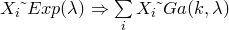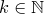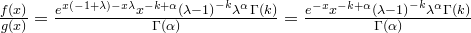# In search of a random gamma variate…

March 16, 2010
By

Want to share your content on R-bloggers? click here if you have a blog, or here if you don't.

One of the most common exersices given to Statistical Computing,Simulation or relevant classes is the generation of random numbers from a gamma distribution. At first this might seem straightforward in terms of the lifesaving relation that exponential and gamma random variables share. So, it’s easy to get a gamma random variate using the fact that.

The code to do this is the following

```rexp1 <- function(lambda, n) {
u <- runif(n)
x <- -log(u)/lambda
}

rgamma1 <- function(k, lambda) {
sum(rexp1(lambda, k))
}```

This works unfortunately only for the case.

In the general case we got to result to more “complex” (?) simulation, hence programming. The first technique we gonna use is rejection sampling. As the proposal (or proxy or instrumental) density we set the. The key to implementation is to maximise the ratio of the two densities, ie.

We find the maximum of the ratio along the next lines.

```m<-function(x) exp(-x)*x^(-k+a)*(1/(-1+r))^k*(r^a)*gamma(k)/gamma(a)
grid<-seq(0,10,by=.1)
a=3.45
k=3
r=2.06
plot(m(grid)~grid,type="l",col="red")
grid()
ind<-which.max(m(grid))
# 6
m.max<-grid[ind]
# 0.5```

Analytically we can work out that the maximum is achieved at \$latex alpha -k\$, then the actual value is.Now, we draw variates from the integer gamma until one is accepted.

UPDATED

```n=200000
a=3.45
k=3
r=2.06
lambda=2.71

sample<-rep(NA,n)

start<-Sys.time()
for (i in 1:n) {
# The following is a function tha draws ONE random variate.
# It's useful to have it in this form
one <- function(a, lambda) {
k <- floor(a)
m <-m(a-k)
while (TRUE) {
x <- rgamma1(k,lambda-1)
p.accept <- dgamma(x,a,lambda)/(m*dgamma(x,k,lambda-1))
if (runif(1)
# Time difference of 25.738 secs

grid2 <- seq(0, 10, length.out=100)
plot(grid2, m(a-k)*dgamma(grid2,k,lambda-1), type="l", lty=2, col="red",
xlab="x", ylab="Density",lwd=2)
lines(grid2, dgamma(grid2,a,lambda), col="green",lwd=2)
lines(density(sample),col="steelblue",lwd=2)
legend("topright", col=c("red","green","steelblue"),lty=c(2,1),
legend=c("m(a-k)*g(x)","sample dansity","f(x)"))
```R-bloggers.com offers daily e-mail updates about R news and tutorials about learning R and many other topics. Click here if you're looking to post or find an R/data-science job.
Want to share your content on R-bloggers? click here if you have a blog, or here if you don't.

Tags: , , , , , , , ,